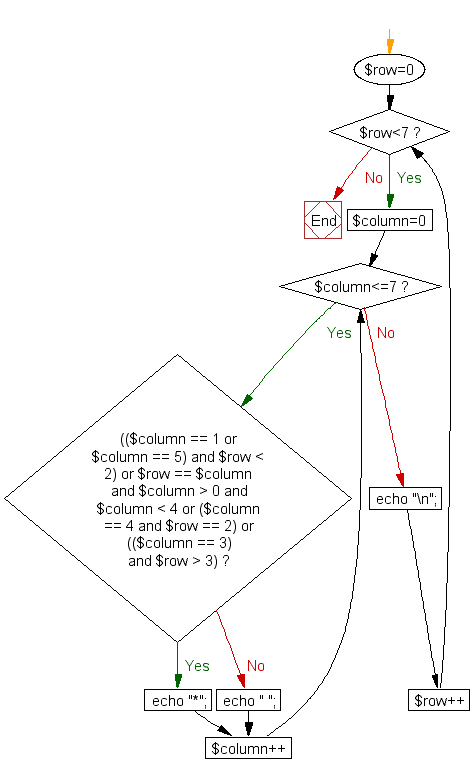﻿ PHP for loops Exercise: Print alphabet pattern Y - w3resource# PHP for loop Exercises: Print alphabet pattern Y

## PHP for loop: Exercise-37 with Solution

Write a PHP program to print alphabet pattern 'Y'.

Pictorial Presentation:Sample Solution:

PHP Code:

``````<?php
for (\$row=0; \$row<7; \$row++)
{
for (\$column=0; \$column<=7; \$column++)
{
if (((\$column == 1 or \$column == 5) and \$row < 2) or \$row == \$column and \$column > 0 and \$column < 4 or (\$column == 4 and \$row == 2) or ((\$column == 3) and \$row > 3))
echo "*";
else
echo " ";
}
echo "\n";
}
?>
```
```

Sample Output:

``` *   *
*   *
* *
*
*
*
*
```

Flowchart :PHP Code Editor:

Have another way to solve this solution? Contribute your code (and comments) through Disqus.

What is the difficulty level of this exercise?

﻿

## PHP: Tips of the Day

PHP: php execute a background process.

Assuming this is running on a Linux machine, I've always handled it like this:

`exec(sprintf("%s > %s 2>&1 & echo \$! >> %s", \$cmd, \$outputfile, \$pidfile));`

This launches the command \$cmd, redirects the command output to \$outputfile, and writes the process id to \$pidfile.

That lets you easily monitor what the process is doing and if it's still running.

```function isRunning(\$pid){
try{
\$result = shell_exec(sprintf("ps %d", \$pid));
if( count(preg_split("/\n/", \$result)) > 2){
return true;
}
}catch(Exception \$e){}

return false;
}
```

Ref : https://bit.ly/35aGQiK